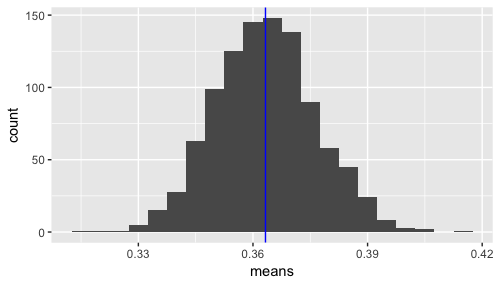# Markov Chain Simulation

I’ve been reading up on Markov chains and related concepts. On the wikipedia page there is an example of a 2 state Markov process. I decided to simulate it in R and plot the mean of the means.

Quick Code example here:The mean of means (of state e) is close to .36. If you take .3 * .36 + .4 * (1-.36), you get .364, so this seems to make sense. Note that I’m weighting the switching to e percentage based on the percentage of being in that state in the first place.

# Simulating the Monty Hall Problem in R.

The Monty Hall Problem is famous in the world of statistics and probability. For those struggling with the intuition, simulating the problem is a great way to get at the answer. Randomly choose a door for the prize, randomly choose a door for the user to pick first, play out Monty’s role as host, and then show the results of both strategies.

The numeric output will vary, but look something like:

```> print(summary(games\$strategy) / nrow(games)) stay switch 0.342 0.658 ```

The following code does this in a rather short R example: